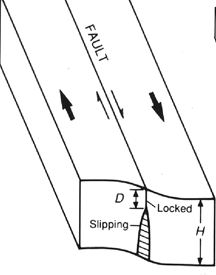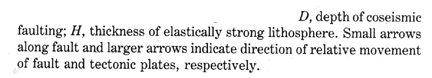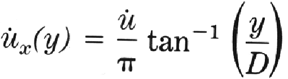# Simple Programming in Matlab

The following lecture and demonstration of scripts and functions illustrate the real power of Matlab and the opportunity to get it to do some work for you. You can create these files in the text editor. They should be saved with a .m extension, and then run by typing their name (and adding arguements in the case of functions), but without the .m at the end.

## Matlab scripts

One thing that you might have realized from your work in the last two weeks is that there can often be a lot of retyping of your work in order to account for some small changes. One powerful aspect of Matlab is the ability to write scripts, which are essentially a series of Matlab commands strung together into a file, the name of which is typed and the commands are executed in series.

Here is a simple script that will say Hello:
```%simple script to say hello world hello.m
fprintf('HELLO WORLD!\n');
```
and how I ran it and the outcome:
```>> hello
HELLO WORLD!
```
The function fprintf can be pretty helpful:

fprintf is a Matlab function that can print a formatted text string to a file or the screen:
```>> fprintf('here is a number = %.3f (that was the formatting)\n',2.5)
here is a number = 2.500 (that was the formatting)
```

The main points are that you put a formatted placeholder in the line (%.3f) which in this case says to format the number as a 3 decimal place floating point. The \n tells Matlab to start a new line. Note the newline and the formatting placeholder along with the text are in the quotes. Then the number follows and is placed where it should be. If you have more than one placeholder, Matlab puts the numbers in sequentially and you can actually evaluate an expression there:

```>> fprintf('here is a number = %.3f (that was the formatting), and here is its half = %.\n',2.5,2.5/2)
here is a number = 2.500 (that was the formatting), and here is its half = 1.250
```
Video explanation of simple script and fprintf

## Matlab functions

"When you use Matlab functions such as inv, abs, angle, and sqrt, Matlab takes the variables that you pass it, computes the required results using your input, and then passes those results back to you. The commands evaluated by the function as well as any intermediate variables created by those commands are hidden. All you see is what goes in and what comes out (i.e., a function is a black box)."--p. 143 from Mastering Matlab 5.

The main differences between a SCRIPT and FUNCTION:
functions scripts
can take input arguments no input
ONLY work with variables created WITHIN the function
or PASSED to the function as input arguments
(functions have their OWN workspace)
operate on any variables within the Matlab workspace
all variables EXCEPT output arguments defined by the function
are NOT retained in the Matlab workspace
after completion
any variables created throughout the script stay in the
Matlab workspace until cleared by the user

### Example of a simple function:

```function r = DegreesToRadians(degrees)
%this function takes an input angle in degrees and converts it to
%JRA, 11.22.99

r = degrees * (pi/180);
```
Here is some Matlab action associated with it:
```>> ls

ans =

this function takes an input angle in degrees and converts it to

ans =

3.1416
```
Video explanation of simple function

Video explanation of function help and function vs. script

### Plot XY function

Making an XY plot is a common Matlab activity. Let's make a function to do it.

### Another example of a simple function (buried slip along a fault)

A very simple model of a plate boundary, vertical strike-slip fault has a locked portion to some depth D and then steady sliding below that "brittle-ductile" transition (Thatcher, 1990):You may recall this equation from your quiz today:It gives the fault parallel velocity (UdotXY) as a function of distance from the fault (located at 0) Y, the depth of locking D, and the long term or deep slip rate Udot.
Let's make a function and plot it.:

Essential Elements for Constructing a Function:
1. First line: function declaration line
2. ```	function output = FunctionName(input);
```
3. Help information: the very next part needs to be a comment section. It will be displayed when you type:
4. ```	help FunctionName
```
Remember to include:
• Name of the function
• Description of what the function does
• Define the input arguments
• Define the output arguments
• An example of how it is used (optional)
• Author's Name
5. Commands to run within the function
6. You MUST define the output argument as a variable in the function
Be sure to look at HERE for the rules for constructing a Matlab function.

NOTE: you have to be in the directory in which the function is or type its complete path or have the path to that directory added to your Matlab search path